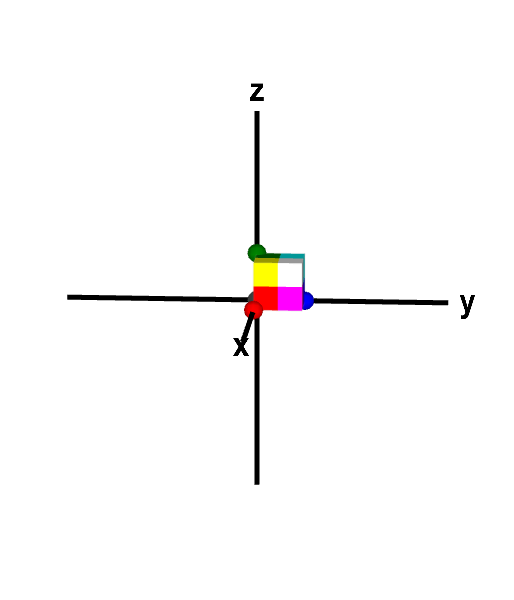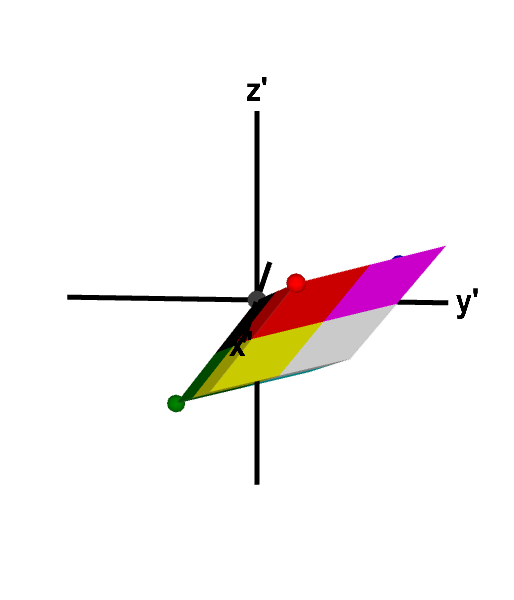# Math Insight

### Applet: A three-dimensional linear transformation that reverses orientationThe linear transformation $\vc{T}=B\vc{x}$, with $$B=\left[\begin{array}{rrr}3&1&-3\\1&3&-2\\1&1&-3\end{array}\right]$$ maps the unit cube to a parallelepiped of volume 14. The expansion of volume is reflected by the determinant $\det B = -14$. Since $\det B$ is negative, $\vc{T}$ not only expands volume by a factor of 14 but also reverse orientation, i.e., reflects objects into their mirror image. The reversal of orientation can be observed through the order of the colors on corresponding faces of the cube and parallelepiped. For example, the cube has a face with the colors ordered black, red, magenta, blue, when moving counterclockwise, while the parallelepiped has a face with the counterclockwise color order black, blue, magenta, red. You can further explore the mapping by changing either shape to other parallelepipeds by dragging the points on four of its vertices.